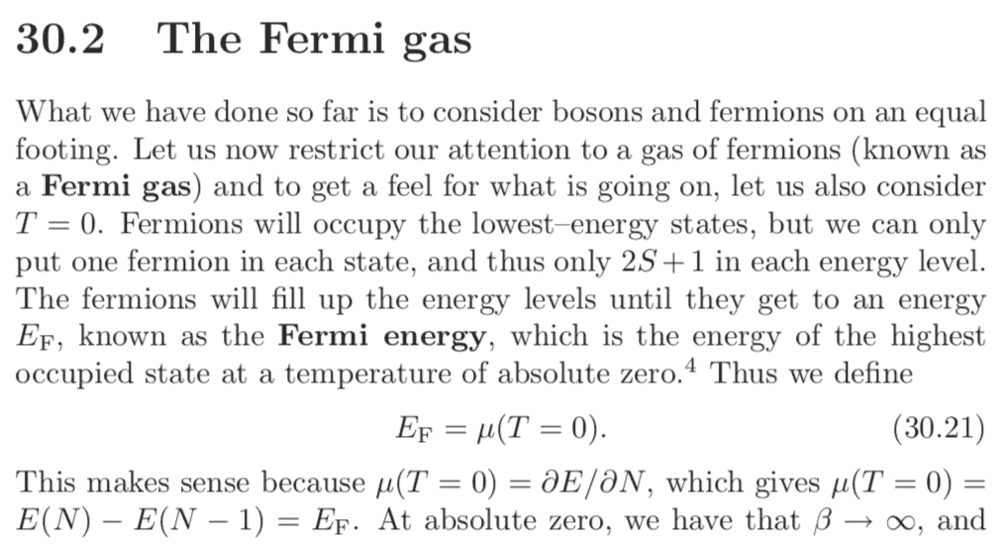# I Thermal Physics: Fermi Gas and chemical potential

#### WWCY

Hi all, I have an issue trying to understand the following paragraph from Blundell's book.How, exactly, does the definition of $\mu_0 = E_F$ "make sense"? In the sentence after 30.21, it seems to say that the mean energy for a system with $N$ particles differs from that of a system with $N-1$ particles by the highest occupy-able energy level at $T = 0$, which is $E_f$. What does this mean?

Many thanks in advance.

#### Attachments

• 304.9 KB Views: 48

#### DrClaude

Mentor
The chemical potential can be seen as the (Gibbs free) energy per particle, or the energy necessary to add one more particle to the system. With fermions at T = 0, all states up to $E_F$ are occupied, therefore the next fermion will add an energy of $E_F$ to the system. It does make sense to equate $\mu(T=0) = E_F$.

•WWCY

### The Physics Forums Way

We Value Quality
• Topics based on mainstream science
• Proper English grammar and spelling
We Value Civility
• Positive and compassionate attitudes
• Patience while debating
We Value Productivity
• Disciplined to remain on-topic
• Recognition of own weaknesses
• Solo and co-op problem solving